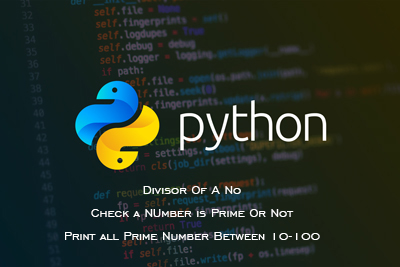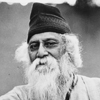FREE ASSISTANCE FOR THE INQUISITIVE PEOPLE
Tutorial Topics
Sum of Digits, Reverse A Numbers, Check Palindrome Number Or Not Fibonacci Sequence
Divisor, Check Prime Numbers - Python Language
304    Arnab De    19/09/2020### Write a python program to find the divisor of A Number.

Each and every number can divide by the 1 and itself. Here we find the other divisor of the nnumber. Now a number can divide by any number which is less than or equal of the half of the number.

```no=int(input("Enter A Number : "))
for i in range(2,int(no/2)+1):
if(no%i==0):
print(i)
```

### Write a python program to check a number is prime or not.

Each and every number can divide by the 1 and itself. But some numbers are not divided by any other number except 1 and it self. Those numbers are known as Prime Numbers. Here we find the divisor the number. if a number has no divisor except 1 and itself then we can say that number is a prime number.

```no=int(input("Enter A Number : "))
fl=1
for i in range(2,int(no/2)+1):
if(no%i==0):
fl=0
break
if(fl==1):
print(no," is a prime no")
else:
print(no," is not a prime no")
```

### Write a python program to print all prime numbers from 10 to 100.

```for no in range(10,101):
fl=1
for i in range(2,int(no/2)+1):

if(no%i==0):
fl=0
break
if(fl==1):
print(no,", " ,end='')
```
Sum of Digits, Reverse A Numbers, Check Palindrome Number Or Not Fibonacci Sequence
Author DetailsArnab De
I have over 16 years of experience working as an IT professional, ranging from teaching at my own institute to being a computer faculty at different leading institute across Kolkata. I also work as a web developer and designer, having worked for renowned companies and brand. Through tutorialathome, I wish to share my years of knowledge with the readers.
Enter New Comment
Comment History
No Comment Found Yet.It is very simple to be happy, but it is very difficult to be simple.
Rabindranath Tagore
440
23.83
Today So Far
Total View (Lakh)